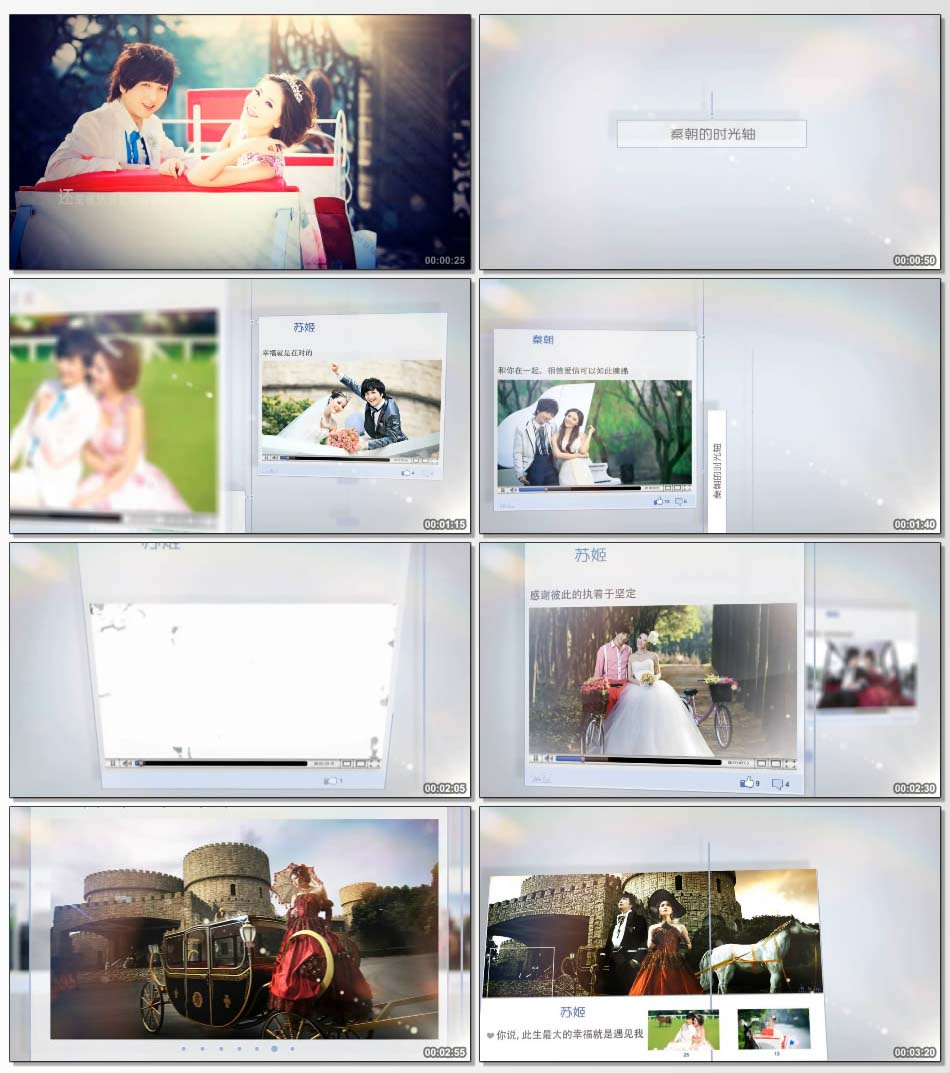# 爱情时间轴t1=你要相信

t2=无论你此时正被光芒环绕被掌声淹没
t3=还是当你正孤独地

t4=无论是飘着

t5=还是被热浪炙烤的黄昏
t6=他一定会穿越这个世界上

t7=他一一的走过他们，走向你
t8=他一定会找到你

t9=我们的时光轴故事
t10=秦朝
t11=苏姬
t12=我们相遇了
t13=我终于找到了他
t14=自从遇见了你，我的生活开始改变
t15=缘分有时候，就是这么奇妙
t16=一路走来，幸福来之不易
t17=幸福就是在对的时间遇见对的人
t18=你就是我要的幸福
t19=和你在一起，发现幸福可以如此简单
t20=和你在一起，相信爱情可以如此缠绵
t21=和你在一起，原来奋斗可以如此开心
t22=和你在一起，感觉回忆可以如此甜美
t23=因为有你，我不再孤单
t24=因为有你，我变的无比坚强
t25=因为有你，我的生活充满无数欢乐
t26=因为有你，我的小世界充满色彩
t27=我们的故事虽然短暂
t28=我们的故事虽然很简单
t29=但那一定是我们追求的幸福
t30=感谢你一直陪伴在我身边
t31=感谢你一直对我的包容
t32=感谢彼此的执着于坚定
t33=才有我们今天的十指相扣，永远在一起
t34=一起的印记，一辈子的旅程
t35=我想，愿得一人心，白首不离
t36=你说，此生最大的幸福就是遇见我
t37=2015.12.18
t38=爱继续...
t39=直到永远
t40=一段情，两个人

t41=三个字就是我爱你

t42=这一天，我为你戴上幸福的戒指

t43=这一世，把你捧在手心里

t44=因为这是我一生的幸福